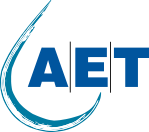# How do tube settler work – plate settler, lamella clarifier tutorial

Tube settlers which are also known as plate settlers or lamella clarifiers are used to increase settling performance within a sedimentation basin. Tube settlers consist of inclined plates or channels that provide a settling surface area for particles in wastewater and drinking water. The total settling area is the sum of the projected area in horizontal direction multiplied with the total amount of plates or channels.

To explain how tube settlers are designed lets take a look at the following example: A drinking water plant has a monthly maximum flow of 1MGD or 3,785m3/day. The amount of total suspended solids entering the plant is 200mg/l and the effluent permit requires 30mg/l. At first, we have to determine the settling velocity of the particles. The settling velocity of the particles very much depends on their size and shape. In general, smaller particles settle slower, hence they have a lower settling velocity. Particles which are smaller than 25 Micrometer can usually not be settled down at all because drag forces (turbulences, rising particles and others) within the sedimentation basin are bigger than the particle settling force. Also, spherical shaped particles settle better than flat shaped particles as their form offers less contact area for drag forces.

Stokes law is a good basis to calculate the settling velocity of particles. The formula: vs settling velocity of the particle has to be bigger as all drag forces  ) calculates the drag force depending on the density delta of the fluid and the particle, the size and form of the particle as well as the laminar flow in the tank.

In our example we have mostly sand and gravel that needs to be removed. The minimum particle size is 50 Micrometer and the density delta between the water and the particles shall be 12.5lbs/ft3 or 200kg/m3. Sand and gravel have a rounded shape therefore we will use a form factor of 0.8. The resulting settling velocity has to be bigger as 1.67m/h or 5.49ft/h.

As stokes law assumes laminar flow, no other colliding particles and other factors but we want to design a sedimentation tank we have to consider an additional safety factor. Depending on the retention time and water turbidity different safety factors can be applied but we will just use a safety factor of 2. Therefore, the resulting settling velocity of the particles is 1.67m/h divided by 2 equals 0.835m/h or 2.75ft/h.

Knowing the settling velocity, we can now determine the total required amount of tube settlers. AET LLC offers different types of tube settlers but for drinking water applications the LS50 design is usually used. This tube settler type has a channel distance of about 1.75in or 45mm and a projected surface area of 3.3 to 3,5ft2/ft3 (11 to 11.5m2/m3). Now to determine the total required amount of tube settlers the settling velocity of the particles has to be bigger as the total projected surface area of the tube settlers divided by the water flow. Solving the equation to x results in a total required projected surface area of 2,046ft2 or 189m2. Dividing this result by 3.3ft2/ft3 equals the total required amount of 623ft3 or 17.2m3 of tube settlers for this application.